# Question: Foundations of Materials Science and Engineering (5th Edition) – Free Chegg Question Answer

Foundations of Materials Science and Engineering (5th Edition)

`Transcribed text From Image:`

`Answer:`

### Problem

In Fig. 8.12, determine the degree of freedom, F, according to Gibbs rule at the following points:

(a) At the melting point of pure tin.

(b) Inside the α region.

(c) Inside the α + liquid region

(d) Inside the α + β region

(e) At the eutectic point

Figure 8.12 The lead-tin equilibrium phase diagram. This diagram is characterized by the limited solid solubility of each terminal phase (α and β). The eutectic invariant reaction at 61.9% Sn and 183°C is the most important feature of this system. At the eutectic point, α (19.2% Sn), β (97.5% Sn), and liquid (61.9% Sn) can coexist.

### Step-by-step solution

1. Step 1 of 1 The equation for Gibbs phase rule for binary phase diagrams is,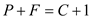…… (1) Here, the number of phases that coexist in the chosen system is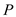, degrees of freedom is F, number of the components in the system is C At the melting point of pure tin: At the melting point of pure tin, number of components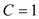, but the number of phases are=2 (solid and liquid). Substitute these values in the equation (1)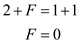Therefore, the degree of freedom is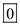. Inside the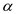region: Number of components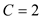, but the number of phases is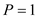(solid). Substitute these values in the equation (1)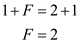Therefore, the degree of freedom is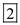. Inside the+ liquid region: Number of components, but the number of phases is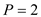(solid and liquid) Substitute these values in the equation (1)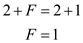Therefore, the degree of freedom is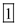. Inside the+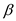region: Number of components, but the number of phases is(2 numbers solid) Substitute these values in the equation (1)Therefore, the degree of freedom is. At the eutectic point: Number of components, but the number of phases is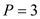(1 number of liquid & two numbers of solid) Substitute these values in the equation (1)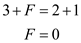Therefore, the degree of freedom is